# CASSCF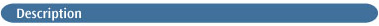This method keyword requests a Complete Active Space Multiconfiguration SCF (MC-SCF) [97,98,137,138,195,405]. An MC-SCF calculation is a combination of an SCF computation with a full CI involving a subset of the orbitals; this subset is known as the active space. The number of electrons (N) and the number of orbitals (M) in the active space for a CASSCF must be specified following the keyword: CASSCF(N,M). Note that options may be interspersed with N and M in any order.

By default, the active space is defined assuming that the electrons come from the highest occupied orbitals in the initial guess determinant and that the remaining orbitals required for the active space come from the lowest virtuals of the initial guess. Thus, for a 4-electron, 6-orbital CAS—specified as CASSCF(4,6)—on a closed-shell system, the active space would consist of:

• Enough occupied orbitals from the guess to provide 4 electrons. Thus, the 2 highest occupied      MOs would be included.

• Enough virtual orbitals to make a total of 6 orbitals. Since 2 occupied orbitals were included,      the lowest 4 virtual orbitals would become part of the active space.

Similarly, a 4 electron, 6 orbital CAS on a triplet would include the highest 3 occupied orbitals (one of which is doubly occupied and two singly occupied in the guess determinant) and the lowest 3 virtual orbitals. In Gaussian 03, algorithmic improvements make an active space of up to about 14 orbitals feasible [99,100,102]. Above 8 orbitals, the CASSCF code automatically uses this new direct method for matrix elements.

Normally, Guess=Alter or Guess=Permute is necessary to ensure that the orbitals which are selected involve the electrons of interest and that they are correlated correctly. A prior run with Guess=Only can be used to quickly determine the orbital symmetries (see the first example below). Alternatively, a full Hartree-Fock single point calculation may be done, and the subsequent job will include Guess=(Read,Permute) in order to retrieve and then modify the computed initial guess from the checkpoint file. You need to include Pop=Regular in the route section of the preliminary job in order to include the orbital coefficient information in the output (use Pop=Full for cases where you need to examine more than just the few lowest virtual orbitals). Alternatively, you may use Pop=NBOSave to save the NBOs, which are often the best choice for starting CAS orbitals. You may also choose to view the orbitals in a visualization package such as GaussView 3.0.

By default, CASSCF calculations use a direct algorithm to avoid disk storage of integrals. A conventional algorithm may be selected by including SCF=Conven in the route section.

CAS is a synonym for CASSCF.

Use #P in the route section to include the final eigenvalues and eigenvectors in addition to the energy and one-electron density matrix in the CASSCF output.

A brief overview of the CASSCF method is given in chapter 9 (exercises 5 and 6) and appendix A of Exploring Chemistry with Electronic Structure Methods, 2nd ed. . See reference  for a detailed discussion on the choice of an active space. See this page for a discussion of efficiency considerations for CASSCF calculations.

Note: CASSCF is a powerful but advanced method with many subtleties. We strongly recommend that you study the cited references before attempting to run production CASSCF calculations (this is especially true for CASSCF MP2). Example applications are discussed in references [406,407,408,409,410,411,412].

### VARIATIONS

• An MP2-level electron correlation correction to the CASSCF energy may be computed during      a CASSCF calculation by specifying the MP2 keyword in addition to CASSCF within the route section .

• Calculations on excited states of molecular systems may be requested using the NRoot option.     Note that a value of 1 specifies the ground state, not the first excited state (in contrast to usage with the CIS keyword).

• State-averaged CASSCF calculations may be performed using the StateAverage and NRoot options to specify the states to be used.

• Conical intersections and avoided crossings may be computed by including Opt=Conical in the route section of a CASSCF job (see the examples) [165,166,167].

• Approximate spin orbit coupling between two spin states can be computed during CASSCF calculations by including the SpinOrbit option [250,251,252,253,254,413,414]. The method used in Gaussian 03 is based on reference . It is available for the elements H through Cl.

In order to compute the spin orbit coupling, the integrals are computed in a one-electron approximation involving relativistic terms, and then effective charges are used that scale the Z value for each atom to empirically account for 2 electron effects. This value can be specified for each atom via the molecule specification nuclear parameters list.

Finally, note that such calculations will be state-averaged by default.

• The Restricted Active Space variation (RASSCF)  is now supported . It is selected via the RAS option. RASSCF calculations partition the molecular orbitals into five sections: the lowest lying occupieds (doubly occupied in all configurations), the RAS1 space of doubly occupied MOs, the RAS2 space containing the most important orbitals for the problem, the RAS3 space of weakly occupied MOs and the remaining unoccupied orbitals. Thus, the active space in CASSCF calculations is divided into three parts in a RAS calculations, and allowed configurations are defined by specifying the minimum number of electrons that must be present in the RAS1 space and the maximum number that may be in the RAS3 space, in addition to the total number of electrons in the three RAS spaces. See the discussion of the RAS option for the methods for specifying these values.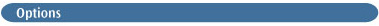NRoot=j
Requests that the jth root of the CI be used, so that an excited state is obtained when j > 1. The option defaults to the ground state (j=1). The state specified by NRoot is referred to as the "state of interest."

StateAverage
Used to specify a state-averaged CASSCF calculation. All states up to NRoot are averaged. This option requires the weighting for the various states to be input in format nF10.8 (no trailing blank line). StateAverage is not allowed in combination with Opt=Conical or CASSCF=SpinOrbit, both of which perform state-averaged calculations by default.

SpinOrbit
Compute approximate spin orbit coupling between two states, specified on a separate input line. Implies a state-averaged CASSCF calculation.

RAS=(a,b,c,d)
Requests a RASSCF calculation which allows up to a holes (i.e., excitations from RAS1 into RAS2 or RAS3) in the b orbitals in the RAS1 space, and to c particles in the d orbitals in the RAS3 space (i.e., excitations from RAS1 or RAS2 into RAS3). Thus, the minimum number of electrons in RAS2 is 2b-a. Note that the two CASSCF keyword parameters specify the size of the entire active space: RAS1 + RAS2 + RAS3 (see the examples).

DavidsonDiag
Requests the use of the Davidson diagonalization method for the CI matrix instead of the Lanczos iterations. Lanczos is the default for NRoot values of 1 or 2; otherwise, Davidson is the default.

FullDiag
Requests the use of the full (Jacobi) diagonalization method for the CI matrix instead of Lanczos or Davidson iterations. The default is full diagonalization if there are 6 or fewer active orbitals, and Davidson otherwise. NoFullDiag suppresses the use of the full diagonalization method.

The full Jacobi diagonalization method must be used if quadratic convergence is required (see the QC option below), and when one knows nothing at all about the CI eigenvector (in the latter case, specify FullDiag for calculations involving more than 6 active orbitals)

StateGuess=k
Set the starting vector for the Lanczos method to configuration k. For example, this option can be useful for selecting a configuration of the correct symmetry for a desired excited state (different from that of the ground state). In such cases, running a preliminary calculation to determine the orbital symmetries may be required.

k may also be set to the special value Read, which says to read in the entire eigenvector from the input stream (format: NZ, (Ind(I), C(Ind(I)), I=1, NZ).

The default diagonalization method is most efficient if the size of the CI problem is greater than about 50, or the user can identify one or more dominant components in the eigenvector from the onset of the calculation, via the initial trail vector. By default, the starting vector is initialized in j+1 positions, where j is the value given to the NRoot option (or its default value). The positions correspond to the lowest j+1 energy diagonal elements of the CI Hamiltonian. This usually results in good convergence for the lowest j roots.

The StateGuess option (below) may be used to change this default. CASSCF(…,StateGuess=k) sets C(k) to 1.0. The central requirement for this vector is that it not be deficient in the eigenvector that is required. Thus, if the CI eigenvector is dominated by configuration k, setting the StateGuess option to k will generate a good starting vector (e.g., StateGuess=1 is appropriate if the CI vector is dominated by the SCF wavefunction). However, if the coefficient of configuration k is exactly zero (e.g., by symmetry) in the desired root, then that eigenvector will be missing, and the calculation will converge to a higher state.

OrbRot
OrbRot includes and NoCPMCSCF excludes the orbital rotation derivative contributions from the CP-MC-SCF equations in an Opt=Conical calculation. OrbRot is the default.

SlaterDet
Use Slater determinants in the CASSCF calculation. This option is needed to locate a conical intersection/avoided crossing between a singlet state and a triplet state.

HWDet
Use Hartree-Waller determinants instead of Slater. This is the default for CAS calculations involving 10 or more orbitals. It implies NoFullDiag.

RFO
Requests the RFO quadratic step. At most, one of QC and RFO should be specified.

QC
Requests a quadratically convergent algorithm for the CAS. This option should be used with caution; it works well only with a very good guess. Only one of QC and RFO should be specified.

UNO
Requests that the initial orbitals for the CAS be produced from the natural orbitals generated from a previous UHF calculation [415,416]. Normally used with Guess=Read.

The UNO guess must be used with caution. Often, some of the natural orbitals which have modest occupation are not the important ones for the process of interest. Consequently, unless the entire valence space is being correlated (which is usually prohibitively expensive), one normally runs one job which does a UHF calculation with Pop=NaturalOrbital, and then examines the resulting orbitals. The orbitals which belong in the active space are then selected, and a single-point CASSCF(…,UNO) Guess=(Read, Alter) calculation is performed. The resulting converged orbitals are then examined to verify that the correct active space has been located, and finally an optimization can be run with CASSCF(…,UNO) Guess=Read. For singlets, this entire process depends on the user being able to coax the UHF wavefunction to converge to the appropriate broken spin-symmetry (non-RHF) result.

NPairs=n
Number of GVB pairs outside of the CAS active space in a CAS-GVB calculation .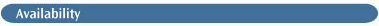Energies, analytic gradients, and analytic and numerical frequencies. CASSCF may not be combined with any semi-empirical method.

Analytic polarizabilities may not be performed with the CASSCF method. Use CASSCF Polar=Numer.

You can restart a CASSCF calculation by specifying SCF=Restart in the route section. In order to restart a CASSCF optimization, the keywords CASSCF Opt=Restart Extralinks=L405 must be included in the job's route section.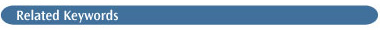Opt=Conical, MP2, Guess, Pop, SCF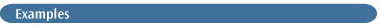We will consider several of the most important uses of the CASSCF method in this section.

Preliminary Examination of the Orbitals (Guess=Only). The following route section illustrates one method of quickly examining the orbitals in order to determine their symmetries and any alterations needed to produce the desired initial state. We include Pop=Reg to obtain the molecular orbital output in the population analysis section:

`# HF/3-21G Guess=Only Pop=Reg Test`

The molecule being investigated is 1,3-cyclobutadiene, a singlet with D2h symmetry. We are going to run a 4x4 CAS, so there will be four orbitals in the active space: 2 occupied and 2 virtual. We want all four orbitals to be π orbitals.

The HOMO is orbital 14; therefore, orbitals 13 through 16 will comprise the active space. When we examine these orbitals, we see that only orbitals 14 and 15 are of the correct type. The molecule lies in the YZ-plane, so π orbitals will have significantly non-zero coefficients in the X direction. Here are the relevant coefficients for orbitals 10 and 13-16:

```Molecular Orbital Coefficients
10          13        14         15        16
O           O         O          V         V
3 1 C   2PX      0.29536    0.00000    0.34716    0.37752   0.00000
7       3PX      0.16911    0.00000    0.21750    0.24339   0.00000
12 2 C   2PX      0.29536    0.00000    0.34716   -0.37752   0.00000
16       3PX      0.16911    0.00000    0.21750   -0.24339   0.00000
21 3 C   2PX      0.29536    0.00000   -0.34716   -0.37752   0.00000
25       3PX      0.16911    0.00000   -0.21750   -0.24339   0.00000
30 4 C   2PX      0.29536    0.00000   -0.34716    0.37752   0.00000
34       3PX      0.16911    0.00000   -0.21750    0.24339   0.00000
```

Orbital 10 is clearly also a π orbital. If we look at higher virtual orbitals, we will find that orbital 19 is also a π orbital. We have found our four necessary orbitals, and can now use Guess=Alter to move them into the active space. Here is the input file for the CASSCF calculation:

```
# CASSCF(4,4)/3-21G Guess=Alter Pop=Reg  Test

1,3-Cyclobutadiene Singlet, D2H, Pi 4x4 CAS

0 1
molecule specification

10,13      Interchange orbitals 10 and 13.
16,19      Interchange orbitals 16 and 19. ```

CASSCF Energy and the One-Electron Density Matrix. When we run this CASSCF calculation on cyclobutadiene, we will obtain a prediction for the energy. It appears in the CASSCF output as follows:

```
TOTAL                   -152.836259 ...  energy at each iteration
ITN=  9 MaxIt= 64 E=  -152.8402786733 DE=-1.17D-05 Acc= 1.00D-05
ITN= 10 MaxIt= 64 E=  -152.8402826495 DE=-3.98D-06 Acc= 1.00D-05
...
DO AN EXTRA-ITERATION FOR FINAL PRINTING ```

The value of E for the final iteration is the predicted energy: -152.8402826495 hartrees in this case.

It is also important to examine the one-electron density matrix, which appears next in the output:

```Final one electron symbolic density matrix:
1             2            3             4
1  0.191842D+01
2 -0.139172D-05  0.182680D+01
3  0.345450D-05  0.130613D-05  0.172679D+00
4  0.327584D-06  0.415187D-05  0.564187D-06  0.820965D-01
MCSCF converged.```

The diagonal elements indicate the approximate occupancies for each successive orbital in the active space. If any of these values is (essentially) zero, then that orbital was empty throughout the calculation; similarly, if any of them is essentially 2, then that orbital was doubly occupied throughout the CAS. In either case, there were no excitations into or out of the orbital in question, and there is probably a problem with the CASSCF calculation. In our case, the two "occupied" orbitals have values less than 2, and the other two orbitals in the active space have non-zero occupancies, so things are fine.

CASSCF MP2 Energy. When you run a CASSCF calculation with correlation (CASSCF MP2 in the route section), the following additional lines will appear in the CASSCF output (with the first one coming significantly before the second):

```MP2 correction to the MCSCF energy is computed     Indicates a CASSCF MP2 job.
...
E2 = -0.2635549296D+00 EUMP2 = -0.15310383973610D+03
Electron correlation-corrected energy.```

The string EUMP2 labels the energy; in this case, the value is -153.1038397361 hartrees.

CAS Configuration Information. The beginning of the CASSCF output lists the configurations, in the following format:

```
PRIMARY BASIS FUNCTION= 1  2  1  2
2                        SYMMETRY TYPE = 0
1    3
1    2
3                        SYMMETRY TYPE = 0
2    3
1    2 ```

The first line indicates the electron assignments for the reference configuration. This is a 4x4 CAS, so the primary basis function output indicates that there is an α and b electron in both orbitals 13 and 14 (the numbers refer to the orbitals in the active space, from lowest to highest, and the electron order in the output is: α α β β). In configuration 2, the α electron in orbital 13 remains there, the α electron from orbital 14 has been excited to orbital 15, the β electron in orbital 13 remains there, as does the β electron in orbital 14. Similarly, in configuration 3, there is a β electron in orbital 13, an α (from 13) and β electron in orbital 14, and an α electron in orbital 15.

Using CASSCF to Study Excited States. The following two-step job illustrates one method for studying excited state systems using the CASSCF method. The first step assumes that a preliminary Hartree-Fock single point calculation has been done in order to examine the orbitals; it takes advantage of the initial guess computation done by that job, which it retrieves from the checkpoint file:

```%chk=CAS1
# CASSCF(2,4) 6-31+G(D) Guess=(Read,Alter) Pop=NaturalOrbital Test
Geom=Check

Alter the guess so that the three LUMOs are all the desired symmetry, and run the CAS

0,1

orbital alterations

--Link1--
%chk=CAS1
%nosave
# CASSCF(2,4,NRoot=2) 6-31+G(D) Guess(Read) Pop(NaturalOrbital)
Geom=Check Test

Excited state calculation

0,1```

The second job step uses the NRoot option to CASSCF to specify the first excited state. The first excitation energy for the system will then be computed by taking the energy difference between the two states (see exercise 5 in chapter 9 of Exploring Chemistry with Electronic Structure Methods  for a more detailed discussion of this technique).

Predicting Conical Intersections. Including Opt=Conical keyword in the route section changes the job from an optimization of the specified state using CASSCF to a search for a conical intersection or avoided crossing involving that state. The optimized structure will be that of the conical intersection or avoided crossing. Distinguishing between these two possibilities may be accomplished by examining the final eigenvalues in the CASSCF output for the final optimization step (it precedes the optimized structure):

```FINAL EIGENVALUES AND EIGENVECTORS
VECTOR EIGENVALUES      CORRESPONDING EIGENVECTOR

state   energy
1    -154.0503161     0.72053292      -0.48879229 ...
-0.16028934E-02   0.31874441E-02 ...
2    -154.0501151     0.45467877       0.77417416 ...```

If the two eigenvalues (the first entry in the lines labelled with a state number) are essentially the same, then the energies of the two states are the same, and it is a conical intersection. Otherwise, it is an avoided crossing.

Spin Orbit Coupling. Here is the output from a CASSCF calculation where the spin orbit coupling has been requested with the Spin option (the coupling is between the state specified to the NRoot option and the next lower state):

```
****************************
spin-orbit coupling program
****************************
Number of configs= 4
1st state is 1     Identifies the two states between which the spin orbit coupling is computed.
2nd state is 2
Transition Spin Density Matrix
1             2
1    .000000D+00   .141313D+01
2    .553225D-01   .000000D+00
magnitude in x-direction=     .0000000 cm-1
magnitude in y-direction=     .0000000 cm-1
magnitude in z-direction=   55.2016070 cm-1
total magnitude=   55.2016070 cm-1           Spin orbit coupling.
MCSCF converged.```

The spin orbit coupling is broken down into X, Y, and Z components, followed by its total magnitude, which in this case is 55.2016070 cm-1.

RASSCF example. Here is an example RASSCF calculation route section:

`# CAS(16,18,RASSCF(1,2,3,4)) 6-31G(d) `

If this molecule is a neutral singlet, then this route defines the following spaces: RAS1 with 2 orbitals, 3 or 4 electrons in all configurations; RAS2 with 12 orbitals, 12 electrons in the reference configuration; and RAS3 with 4 orbitals, 0-3 electrons in all configurations. Thus, the RAS2 space will have 9 to 13 electrons in all configurations. The orbitals taken from the reference determinant for the active space are (assuming a spin singlet) the 8 highest occupieds and 10 lowest virtuals: i.e., same orbitals as for a regular CAS(16,18).